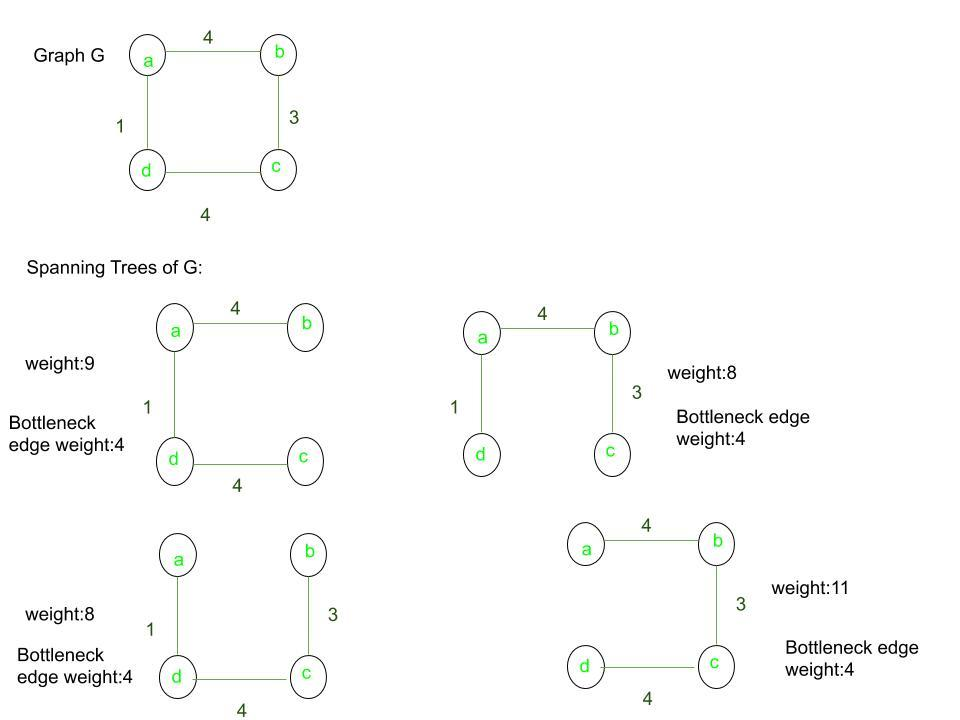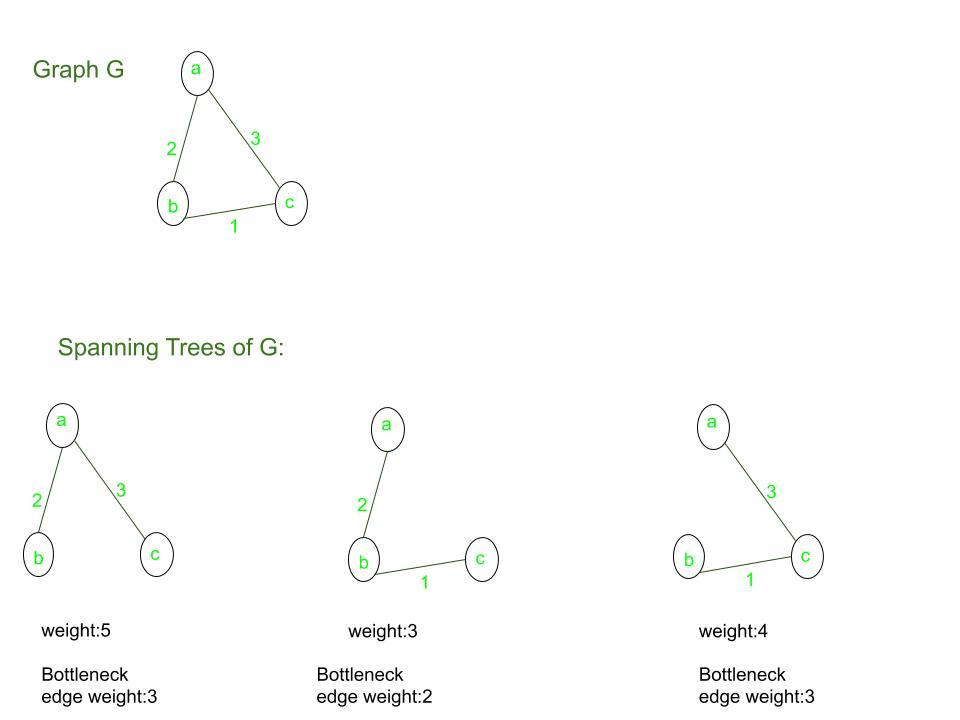# Minimum Bottleneck Spanning Tree(MBST)

The minimum bottleneck spanning tree in an undirected graph is a tree whose most expensive edge is as minimum as possible. In this article, we will understand more about how to identify a minimum bottleneck spanning tree and understand that every minimum spanning tree is a minimum bottleneck spanning tree.

A minimum spanning tree is completely different from a minimum bottleneck spanning tree. A bottleneck in a spanning tree is the maximum weight edge present in the tree. There may be many bottlenecks for the same spanning tree. Let’s understand this with the following examples:

Example 1: Let the given graph be G. Let’s find all the possible spanning trees possible.For the given graph G, the above figure illustrates all the spanning trees for the given graph. Among the spanning trees, the minimum spanning trees are the ones with weight 8. Since all the spanning trees have the same value for the bottleneck edge, all the spanning trees are Minimum Bottleneck Spanning Trees for the given graph. But, all are not minimum spanning trees, since the overall weight is minimum(8) only for the two of the spanning trees.

Example 2: Let the given graph be G. Let’s find all the possible spanning trees possible.For the given graph G, the above figure illustrates all the spanning trees for the given graph. Among the spanning trees, the minimum spanning tree is the one with weight 3. The Minimum Bottleneck Spanning trees for the graph are the trees with bottleneck edge weight 3. Here, the minimum spanning tree is a minimum bottleneck spanning tree but not all minimum bottleneck spanning trees are not minimum spanning trees.

Proof that every Minimum Spanning Tree is a Minimum Bottleneck Spanning Tree: Suppose T be the minimum spanning tree of a graph G(V, E) and T’ be its minimum bottleneck spanning tree. Consider the maximum weight edge of T and T’(bottleneck edge). Then, there are three cases possible:

1. Case 1: If both the edges happen to be the same edge. Then, T is a minimum bottleneck spanning tree (i.e.), every MST is an MBST, due to the arbitrary choice of G.
2. Case 2: If both the edges happen to be different edges, it cannot happen that the weight of T’ is greater than the maximum weighted edge in T since T’ is an MBST.
3. Case 3: Let’s assume that T has a maximum weight edge (p, q) whose weight is greater than the weight of the bottleneck spanning tree T’. Then:
• Let X be the subset of the vertices of V in T that can be reached from p without going through q.
• Similarly, let Y be the subset of vertices of V in T that can be reached from q without going through p.
• Since G is a connected graph, there should be a cut edge between X and Y. The only edge that can be added across this cut is the one of minimum weight.
• But, by the way in which X and Y are defined, we know that (p, q) is the only possible cut edge of minimum weight.
• However, we have a bottleneck spanning tree T’ with lesser weight than w(p, q).
• This is a contradiction because a bottleneck spanning tree itself is a spanning tree and it must have an edge across this cut. And, it will be of lesser weight than w(p, q).
• So, the assumption is wrong and the only possibility is that the maximum weight edge of T and T’(bottleneck edge) are the same.
• Then, by Case 1, the proof has been completed and hence it has been shown that every MST is an MBST.

Attention reader! Don’t stop learning now. Get hold of all the important DSA concepts with the DSA Self Paced Course at a student-friendly price and become industry ready.

My Personal Notes arrow_drop_upCheck out this Author's contributed articles.

If you like GeeksforGeeks and would like to contribute, you can also write an article using contribute.geeksforgeeks.org or mail your article to contribute@geeksforgeeks.org. See your article appearing on the GeeksforGeeks main page and help other Geeks.

Please Improve this article if you find anything incorrect by clicking on the "Improve Article" button below.

Article Tags :
Practice Tags :

Be the First to upvote.

Please write to us at contribute@geeksforgeeks.org to report any issue with the above content.